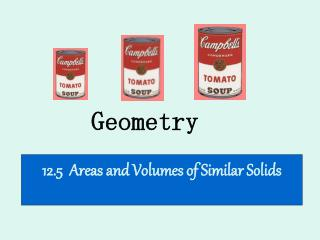DownloadDownload PresentationGeometry

# Geometry

Download Presentation## Geometry

- - - - - - - - - - - - - - - - - - - - - - - - - - - E N D - - - - - - - - - - - - - - - - - - - - - - - - - - -
##### Presentation Transcript

1. Geometry 12.5 Areas and Volumes of Similar Solids

2. Review: Similar Polygons Similar polygons have the same shape but not necessarily the same size. Angles are congruent and sides are proportional.

3. Please turn to your vocab list and add… -Similar solids -Area ratio of similar solids -Volume ratio of similar solids

4. Review: Similar Polygons Regular polygons and circles are always similar to each other. equilateral triangles squares circles regular pentagons The scale factor describes the relationships of the sides or radii.

5. Similar Solids Similar solids have the same shape but not necessarily the same size. Like circles, all spheres are similar.

6. Similar Solids Two solids are similar if and only if their bases are similar and their corresponding lengths are proportional. 6 2 = Scale Factor: 2:3 9 3 4 2 = 15 6 3 10 10 2 6 = 4 15 3 6 9 The bases are similar rectangles because length and width are proportional. The corresponding heights are also proportional.

7. Similar Solids To determine if two solids are similar: (1) Find out if their BASES are similar • regular polygons are always similar • for other polygons, check if sides are • proportional (2) Compute the scale factor (3) Check that the heights are to scale.

8. Are the given solids similar? • Two regular square pyramids have heights 10 and 12. • The bases are squares with sides 4 and 4.8, respectively. All squares are similar. 4 4.0 40 5 10 5 YES = = = = 6 12 6 4.8 4.8 48 2. One rectangular solid has length 7, width 5, and height 3. Another rectangular solid has length 14, width 10, and height 9. Bases are similar. 7 5 1 3 1 Heights are not. NO = = = 14 10 2 9 2 3. Two right triangular prisms have heights 4 and 6. Their bases are triangles with sides 3, 4, 5, and 6,8,10, respectively. Bases are proportional, therefore similar. Heights are not. NO

9. Scale Factor If the scale factor of two solids is a:b, then (1) the ratio of corresponding perimeters is a:b (2) the ratio of base areas, of lateral areas, and of the total area is a²:b² (3) the ratio of volumes is a³:b³ SCALE FACTOR: 1:2 Base circumference: 6π:12π 1:2 Lateral areas: 15π:60π 1:4 Volumes: 12π:96π 1:8 10 8 5 4 • 3 6

10. Exercises Find the missing information. 2:7 5:6 3:10 2:5 2:5 1:9 25:36 9:100 4:25 4:25 1:27 8:343 8:125

11. Exercises 9. Two similar cones have volumes 27 and 64. Find the ratio of: a. the radii b. the slant heights c. the lateral areas 3:4 3:4 9:16 ³√27 = 3 ³√64 = 4

12. Exercises 10. Two spheres have radii 5 cm and 7 cm. Find the ratio of: a. the areas b. the volumes 5²:7² 5³:7³ 25:49 125:343 7 5 Do #11 on your own. Answers: a. 9:49 b. 27:343

13. Exercises 3 2 12. Two foam plastic balls have scale factor 2 : 3. a. If the smaller ball has radius 6 cm, what is the radius of the larger ball? 2 6 2x = 18 = 9 cm. x = 9 3 x b. If the area of the larger ball is 36 cm2, what is the area of the smaller ball? x x 9x = 144π 2² 4 16π cm² = = x = 16π 3² 36π 9 36π c. If the larger ball weighs 12 g, about how much does the smaller ball weigh? (Hint: Weight is related to volume) x x 27x = 96 2³ 8 About 3.6 grams = = x ≈3.6 3³ 12g 27 12

14. Homework pg. 511 WE #1-11 all, 13-19 odd Formula Quiz/Vocab Quiz on Thursday Chapter 12 Test on Friday